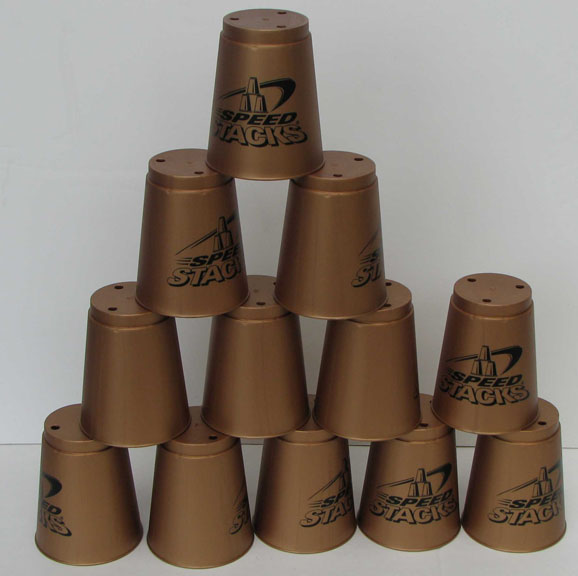# Fastest way to add multiple elements to an Array / Vector in AS3

In a simple situation, where you wish to add many elements to an Array or Vector, you might just do:

```var input:Array;
var output:Array;
while (input.length)
output.push(input.shift());```

However, the sizes of both Arrays are manipulated for each loop, which will have an adverse impact on speed and memory usage. So, we could cache the length of the input Array and not manipulate it:

```var input:Array;
var output:Array;
var inputLength:uint = input.length;
for (var i:int = 0; i < inputLength; i++)
output.push(input[i]);```

But we’re still growing the size of the output Array incrementally, which is very bad. Since we know input.length in advance, we could grow the output Array to its new size just once, before the loop:

```var input:Array;
var inputLength:uint = input.length;
var output:Array;
var outputLength:uint = output.length;
var newOutputLength:uint = outputLength + inputLength;
output.length = newOutputLength;
for (var i:int = 0; i < inputLength; i++)
output[i + outputLength] = input[i]);```

This is OK, but still involves a loop. If only we could push multiple elements into the push method in one go. Well, we can – enter the apply method. Since Array.push accepts multiple arguments (something rarely used) and apply allows us to pass an Array of arguments to any Function, one line and we’re done:

```var input:Array;
var output:Array;
output.push.apply(null, input);```

This works out faster and more memory efficient than the other methods. It works nicely for Vectors, too. If anyone has a faster method of doing this, do let me know.